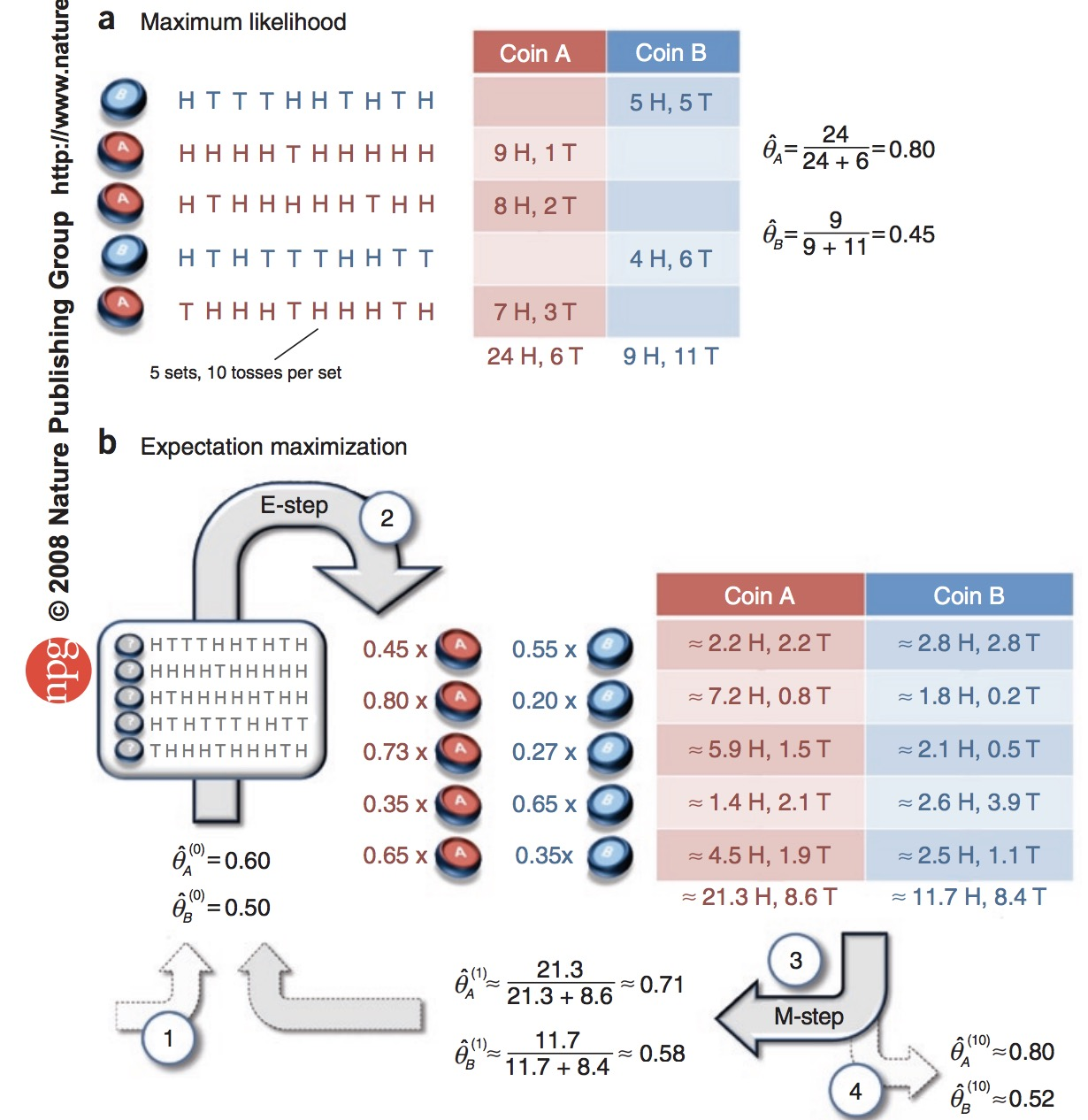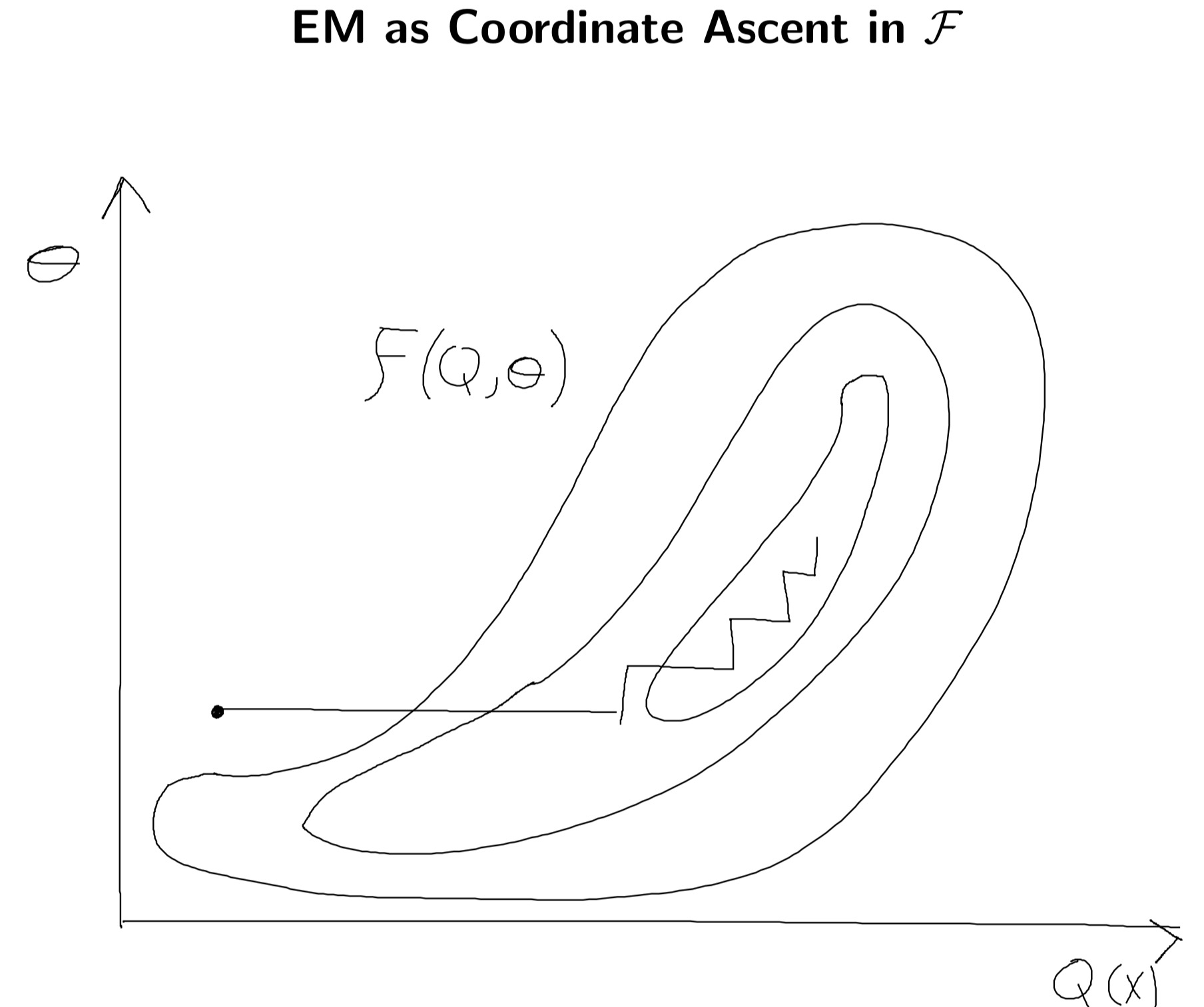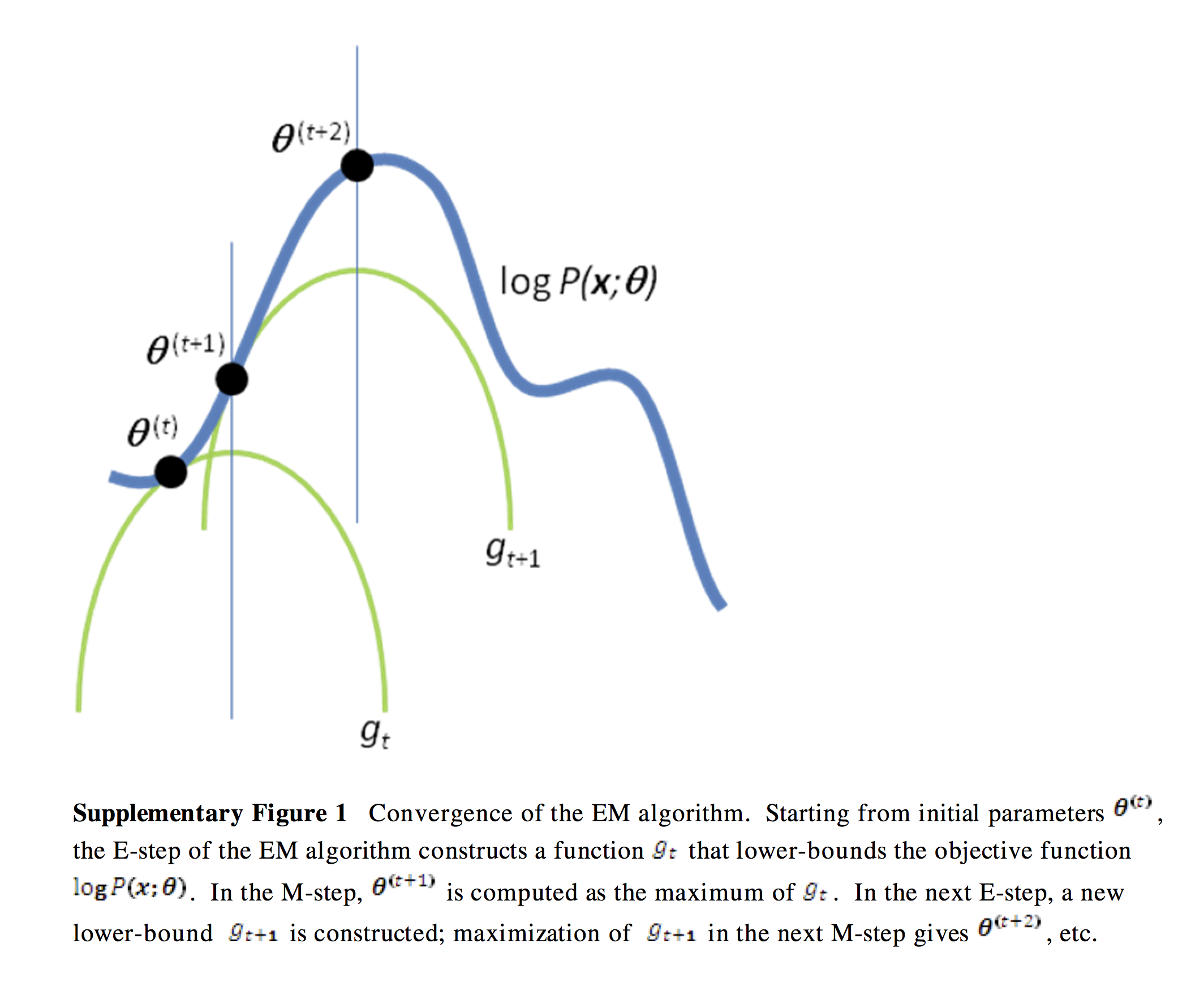Expectation Maximization

Posted by GwanSiu on November 21, 2018

1. Introduction

In this article, I will introduce Expectation Maximization(EM) algorithm. EM algorithm is introduced as early as 1950 by Ceppellini etal, and then it widely applied for parameters estiamtion of incomplete data(known X, unkown Y). Our story begin with maximimum lieklihood of complete data(known X, known Y).

Conventional problem of machine learning is parameters estimation problem. We take an exmaple of fipping coins. Suppose we have two coins A and B with unknown biases, $\theta_{A}$ and $\theta_{B}$ respectively. We set an experiment that we fip the coin repeatly. During the experiment, we fip the coin followed the i.i.d principle and keep track the record of the $X={x_{1},…,x_{N}}$ and $Y={y_{1},…,y_{N}}$, where $N$ is the number of samples, $x_{i}$ denotes the number of head we observed and y is an indicator of identity of coins. we assume these data comes from an underlying distribution $P(X,Y\vert \Theta)$, at this time, we assume bernoulli distribution. Thanks to the bayes’rule: $\displaystyle{p(\Theta\vert X, Y)\frac{p(X,Y\vert\Theta)p(\Theta)}{p(X,Y)}}$, Our goal is estimate the parameters $\Theta$. We can adopt maximize log-likelihood estiamtion(MLE): $\log p(\Theta\vert X, Y)=\log p(X,Y\vert\Theta)$

where $p(y_{i}\vert\Theta)=\alpha_{y_{i}}$.

In practice, we only abtain observed data $X$ and label $Y$ is unkonwn. How to solve this kind of problem. Could we directly apply MLE to this kind of problem?

2. EM Algorithm

2.1 Intuition of EM Algorithm

In this time, we consider a more challenging variant problem that we still we want to estimate baises of two coins A and B, we only has the record $Y$ and do not know about the $Y$, where $Y$ is called hidden parameter or latent factor. Parameter estimated problem with complete data is converted into parameter estimated problem with imcomplete data, the likelihood of data is:

at this time, $\alpha_{i}$ and $\Theta_{\alpha_{i}}$ are unknown. We can see that the MLE is not effective for the problem of imcomplete data due to the sum inside of log. This is a big limitation of MLE and thus we need another method to sovle for imcomplete data problem.

However, if we have some way to know the value of $z$, we can use MLE to inference $\theta$ which is the same as parameter estimation with complete data. Thus, we can reduce parameter estimation for this problem with incomplete data to maximum likelihood estimation with complete data. This is the basic idea of EM algorithm. Note: EM algorithm is proprosed to solve problems with discrete latent factors.

One iterative scheme for obtaining completions caould works as follows: starting from some initial parameters, $\theta^{(t)}$, determine for each of the five sets whether coin $A$ or coin $B$ was more liekly to have generated the observed flips(using the current parameter estimates). Then, assume these completions (that is , guessed coin assignments) to be correct, and apply the regular maximum likelihood estimation procedure to get $\tilde{\theta}^{(t+1)}$. Finally, repeat these two steps until convergence.

The expectation maximization algorithm is a refinement on this basic idea that the expectation maximization algorithm computes probabilities for each possible completion of the missing data, using the current parameters $\widetilde{\theta}^{(t)}$. These probabilities are used to create a weighted training set consisting of all possible completions of the data. Then, a modified version of maximum likelihood estimation that deals with weighted training examples provides new parameter estimates, $\widetilde{\theta}^{(t+1)}$.

The figure below show the procedure of maximum likelihood estimator and expectation maximization algorithm. The figure is from the paper: what is the expectation maximization algorithm?Intuitively, the expectation maximization algorithm alternates between the steps of guessing a probability distribution over completions of missing data given the current model( known as the E-step). and then re-estimating the model parameters using these completions(known as the M-step).

2.2 Expectation Step

Let’s go further step into mathematics of EM algorithm. Our goal is to draw parameters estimation of incomplete data under the framework of MLE of complete data. That is:

we can see that $\log(p(X\vert \Theta))$ is a probability distribution due to $\alpha_{Y}$. Thus, it is hard to directly maximize a probability distribution. An alternative way is to maximize the expectation of $\log(p(X\vert \Theta))$, denote as $Q(\Theta,\Theta^{(t)})$. That is the E-step of EM algorithm:

2.3 Maximization Step

M-step is to re-estimate the hidden parameter and model parameters using complete data likelihood.

Firstly, to obtain the $\alpha_{l}$, we should take the derivative respect to $\alpha_{l}$, with the constraint is $\displaystyle{\sum_{l=1}^{K}\alpha_{l}=1}$. Thus, lagrange function is:

thus, the $\frac{\partial L}{\partial \alpha_{l}}= \frac{1}{\alpha_{l}}\displaystyle{\sum_{i=1}^{N}} p(l\vert x_{i}, \Theta^{(t)})=0$, and we obtain $\alpha_{l}=\displaystyle{\frac{\sum_{i=1}^{N} p(l\vert x_{i}, \Theta^{(t)})}{-\lambda}}$. Due to $\displaystyle{\sum_{l=1}^{K}\alpha_{l}=1}$, we can easily get $\lambda=-N$.

Thus:

2.4 Gaussian Mixture Model

Given $N$ samples $X$ and we assume all data samples come from $K$ underlyding gaussian distribution. Thus, $\Theta={\mu_{1},…,mu_{K}, \Sigma_{1},…,\Sigma_{K}}$. The multivarite gaussian model is:

where $p(l\vert x_{i}, \Theta_{l}^{(t)})=\displaystyle{\frac{p(y_{i}=l)p(x_{i}\vert y_{i}=l)}{\sum_{l}^{K}p(y_{i}=l)p(x_{i}\vert y_{i}=l)}=\frac{\pi_{l}\mathcal{N}(x_{i}\vert \mu_{l},\Sigma_{l})}{\sum_{l=1}^{K}\pi_{l}\mathcal{N}(x_{i}\vert \mu_{l},\Sigma_{l})}}$

to abtain the $\mu$, we only focus on the second part:

and set the detivative with respect with $\mu$ to 0, and we have $\sum_{i}^{N}p(l\vert x_{i}, \Theta^{(t)})(x_{i}-\mu_{l})=0$. Thus, $\displaystyle{\mu_{l}^{new}=\frac{\sum_{i}^{N}p(l\vert x_{i}, \Theta^{(t)})x_{i}}{\sum_{i}^{N}p(l\vert x_{i}, \Theta^{(t)})}}$.

To obtain $\Sigma_{l}^{new}$:

where $N_{l,i}=(x_{i}-\mu)(x_{i}-\mu)^{T}$Take the derivative respect to $\Sigma^{-1}$, and we have:

where $M_{l,i}=\Sigma_{l}-M_{l,i}$ and where $S=\frac{1}{2}\sum_{i=1}^{N}p(l\vert x_{i},\Theta^{(t)})M_{l,i}$. Setting $2S-\text{diag}(S)=0$, which implies that $S=0$. This gives:

and we obtain: $\displaystyle{\Sigma_{l}^{new}=\frac{\sum_{i=1}^{N}p(l\vert x_{i}, \Theta^{(t)})(x_{i}-\mu_{l})^{T}(x_{i}-\mu_{l})}{\sum_{i=1}^{N}p(l\vert x_{i}, \Theta^{(t)})}}$.

Finally, we have:

The procedure of EM for Gaussian Mixtures

1. Initilize the means $\mu_{k}, \Sigma_{k}$ and mixing coefficients $\pi_{k}$, and evaluate the initial value of the log likelihood.

2. E-step Evaluate the responsibilities using the current parameter values

1. M-step. Re-estimate the parameters using the current responsibilities

where

1. Evaluate the log likelihood.

and check for the convergence.

2.5 Another View of EM Algorithm

Given observed variable $x$, unobserved variable(latent variable) $z$ and model paramters $\theta$, what we want is maximize likelihood w.r.t $\theta$:

where we rewrite the marginal for observed variable in terms of an integral over the joint distribution for unobserved variable. Our goal is to find a distribution $q(z)$ such that

where $\mathcal{F}(q,\theta)$ is functional, and is a lower bound on the log likelihood. In detail, the lower bound on the log likelihood:

where $\mathcal{H}(q)=-\int q(z)\log q(z)\mathrm{d}z$ is entropy of $q$. Thus, we can iteratively update:

E-step: maximize $\mathcal{F}(q,\theta)$ w.r.t distribution over hidden variables given the parameters:

M-step: maximize $\mathcal{F}(q,\theta)$ w.r.t the parameters given the hidden distribution:which is equivalent to optimizing the expected complete-data likelihood $p(x,z\vert \theta)$, since the entropy of q(z) does not depend on $\theta$.

Although we focus on the lower bound on the log likelihood, the likelihood is non-decreasing in every iteration:

E-step usually construct the upper bound of loglikelihood, and M-step updates model parameters $\theta$. Usually, EM algorithm converges to a local minimum. Thus, please try more times when you implement EM algorithm.The difference between the log likelihood and the bound:

Thus, the likelihood of variable can be decomposed into evidence bound and KL divergence between $q(z)$ and $p(z\vert x,\theta)$.

GMM is a problem of discrete latent variable. EM algoeirhm is a generalized maximimum likelihood estimation in latent variable models.

Appendix

the property of trace

## \begin{align} \text{tr}(A+B)&=\text{tr}(A)+\text{tr}(B)
\text{tr}(AB)&=\text{tr}(BA)
\text{tr}(AB)&=\sum_{i}x_{i}^{T}Ax_{i} \text{ where }B=\sum_{i}x_{i}x_{i}^{T} \end{align} ##

$\vert A\vert$ inicates the determinant of a matrix, and that $\vert A^{-1}\vert = 1/\vert A \vert$.

Matrix derivative, $\displaystyle{\frac{\partial x^{T}Ax}{x}}=(A+A^{T})x$.

If $A$ is the symmetrix matrix

where $A_{ij}$ is the $i,j$-th cofactor of A. we can see that in log-space

by the definition of the inverse of a matrix. Finally, it can be shown that